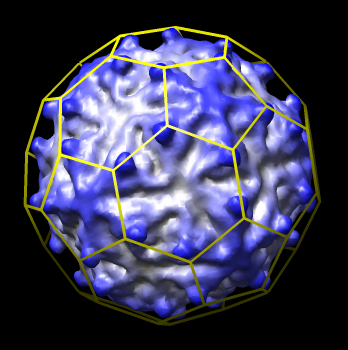# Icosahedral Cage

February 27, 2007

This extension adds a command hkcage that creates a mesh of hexagons and pentagons covering an icosahedron. The number and arrangement of hexagons is governed by two paramters, h and k, and represents ways that proteins are arranged on icosahedral virus capsids.

## Command Syntax

The hkcage command requires 2 arguments and has 5 additional optional arguments

```	hkcage <h> <k> [radius] [orientation] [color-r,g,b,a] [line-thickness] [sphere-factor]
```

The h and k parameters are non-negative integers. The radius gives the distance from the center to an icosahedron 5-fold vertex. The orientation is one of 222 (default), 222r, 2n5, 2n5r, n25, n25r, 2n3, 2n3r and defines the orientation of the icosahedron in the (x,y,z) coordinate space as follows:• 222 - x,y,z axes are 2-fold symmetry axes.
• 222r - same as 222 only rotated 90 degrees about x axis.
• 2n5 - x is a 2-fold symmetry axis, z is a 5-fold symmetry axis.
• 2n5r - same as 2n5r only rotated 90 degrees about x axis.
• n25 - y is a 2-fold symmetry axis, z is a 5-fold symmetry axis.
• n25r - same as n25r only rotated 90 degrees about y axis.
• 2n3 - x is a 2-fold symmetry axis, z is a 3-fold symmetry axis.
• 2n3r - same as 2n3r only rotated 90 degrees about x axis.

The color is specified as 3 or 4 values separated by commas with no spaces red,green,blue,opacity in the range of 0.0 to 1.0 (default is white 1,1,1,1). The line thickness defines the thickness in pixels of the displayed mesh lines (default 1). The sphere factor gives an interpolation factor in the range of 0.0 to 1.0 (default 0) that describes how to interpolate radially between an icosahedron with 20 flat triangular faces and a sphere. A value of 0.0 gives an icoasahedron while 1.0 gives a sphere.

## Technical Notes

Folded hexagons. The individual hexagons are folded at the boundaries between the 20 icosahedron triangular faces.

Straight hexagon edges. The individual hexagon edges are single straight line segments, or two straight light segments when folded across an icosahedron triangle boundary. They do not curve when sphere interpolation is used. Only the hexgon vertex positions are interpolated.

T number. The virus T number is related to parameters h and k by T = h*h + h*k + k*k.

Slow for large h, k. The cage calculation is done in Python and can be slow for large h and k values (15 seconds for h = k = 10 on circa 2006 desktop computer).

## Related Chimera Tool

The Chimera Icosahedron Tool displays a mesh of triangles on an icosahedron. It does not support the hexagonal arrangements defined by the h and k parameters. It is included in Chimera version 1.2186 (November 2005) and later versions and is accessible from menu entry Tools / Higher-Order Structure / Icosahedron Surface.

## Reference

The VIPERdb web site defines how the hexagonal mesh is defined by parameters h and k.

## History

February 27, 2007. Updated for Chimera version 1.2348 which uses NumPy instead of Numeric Python.

October 2, 2006. Created hkcage command for Padmaja Natarajan for use in creating images for VIPERdb web site.